### Compounds

##### Definitions and Asides

Compounds are defined to be multicovers of a single spot by more than just a single polytope. Sure there are infinitely many such possibilities, even continuously related. So the more interesting case comes up, when there is an overall symmetry (not needed to be the same of the used polytopes), under the action of which the total compound is preserved. Sure, there are slightly shifted copies too, which make up such a symmetrical compound: the center of that shift vector serves for inversonal symmetry. Thus, the symmetrical compounds of real interest would require an overall symmetry, which at least is the same (or probably larger) than that of its components. Examples here are all compounds of dual pairs (which by definition have the same overall symmetry as each component – except for selfduals, where symmetry is increased by the exchange of those 2 components) or even the ones given in the lower part of plate 7 (there, in the first 2 cases, componenets are considered under pyritohedral symmetry only, while the compound symmetry comes out to be full cubical).

Compounds moreover are called uniform, if within the definition of polytopal uniformity we replace vertex transivity by vertex figures transivity. This is because for compounds it might be possible to have still no completely coincident elements of subdimension larger than 0, but having coincident vertices none the less. There, this stated demand asks for some symmetry, which interchanges those coincident vertices of the related components as well.

As an aside one might ask whether this uniformity already requires compounds to be isohedral. This can be answered kind of depending: Consider 2 (or more) vertex coincident isohedral compounds (or polytopes), both of the same edge-length, and take the (mere) compound of those. The above stated demand now would require some symmetry which interchanges the vertex figures of the components. This generally would not work. But if we thereafter would identify coincident vertices, the picture changes. We no longer have coincident vertices with single vertex figures each, but we get single vertices with compound vertex figures. Consequently that additional above requirement brakes down, as there is nothing left to be interchanged. (It only remains the question, whether we still have true compounds, or if we would have changed this status, slightly towards mere polytopes, by the application of identification of coinciding vertices.)

Accordingly, more generally we could consider compounds of (possibly) different components, whenever those components have the same circumradius and moreover are inscribed into a common convex hull, while the subset of components of each type already makes up the full overall symmetry of the total compound, and further all those types of components will have the same edge length. (This will brake down in 2D, as different polygons, with the same edge-length, always have different circumradii.) Just to provide examples:

Note that for such uniform compounds (with identified vertices) the demand on uniformity of individual components would not hold! Just consider the (mere) compound of 12 (scaliform) pentagonal pyramids, vertex-inscribed into an icosahedron (which itself will not belong to that compound). That compound thus even qualifies as isohedral. If it additionally is asked to be uniform, from the damand on vertex figure transitivity it becomes clear that vertices need to be identified. – Thus we then constructed a uniform compound (with identified vertices) from non-uniform components! (In fact a hollow and moreover Grünbaumian one, as triagles are completely coincident by 3 each.)

Thus, as long as vertex identification does not take place, uniform compounds need not only be isogonal (by definition), but come out to be isohedral as well. And likewise their components too will be uniform polytopes only.

A compound can be defined to be regular, just like a polytope, when it is transitive on all sub-dimensional elements. Moreover, there are further qualifiers of that type, introduced by Coxeter, vertex-regular and (dually) face-regular (or rather using a corresponding qualifier for the (n-1)-dimensional element). These are achieved, when the vertices (resp. the facet-planes) belong to a regular polytope. That is, when the encasing convex hull (resp. the common intersection kernel) would be a regular polytope. Or, again stated in an other way, vertex-regular compounds occure as facetings of regular polytopes, while face-regular compounds occure as stellations of regular polytopes.

For (isohedral) vertex-regular compounds and their duals Coxeter introduced the following notation:

`a P [b Q] c R`

where Q denotes the (regular) component polytopes (therefore, like P and R too, usually written as Schläfli symbol), b is the count of components. If vertex-regular, one has an encasing regular polytope P, the vertices of which will be used by a components each. Conversely, if face(t)-regular, there is a common intersection kernel which is a further regular polytope R, again possibly with its facet-planes being used by c components each. If vertex-regular but not face(t)-regular, the final part behind the closing square bracket will be omitted; conversely, if face(t)-regular but not vertex-regular the part before the opening one is omitted. (Examples will be given below, as far as applicable. The facet-regular case for Q being a simplex and b=2 will be considered through all dimensions at the end.)

Compounds need some extension to the "normal" incidence matrix description, which is due to their multiple components. The appropriately extended description can be found here, and will be used within the individual compound files linked below.

Some compounds show up an overall symmetry which is the same as that of the individual components too. Then those compounds can be described by a (stacked) Dynkin symbol. Further, compounds of 2 components occasionally might be described as both of the alternated facetings (i.e. compound of snubs). Then they can be described by an holosnub notation.

##### Blends

Closely related with the topics of (generall, i.e. not necessarily uniform) compounds is the topic of blends, a concept intoduced by Olshevsky. Likewise it might deal with multicovers of a single spot by more than one polytope (infact, here the number generally will be 2, asked by the dyadicity argument used below). For blends this furthermore asks for having at least one of its facets each completely coincident. The blend then will be set up by the reduction of the component polytopes by these coincident facets, re-adjoining these reductions dyadically at those coincident (open) ridges. Therefore, blends (of polytopes) are still true polytopes. Also there are 2 kinds of blends, internal and external, depending on having the polytopal centers on the same resp. on different sides of the facetal hyperplane. For instance, many of the Johnson solids are external blends of easier components. (The idea of blends sure can also be applied to compounds instead of mere polytopes.) For an explicit example of an internal blend consider the one built from 2 pentagonal prisms (pip), re-adjoined (internally) at a lacing square, having the componental axes arranged orthogonally (tupip). – The withdrawn original doubled up facets clearly still hold their shapes, just having no body any more, therefore those could be spoken of as pseudo facets.

It further might occur that subsets of uniform polytopes of the same regiment form a group under the action of blending. Such a group then is called a cohort. For instance co, oho, and cho make up such a cohort of 3. (In fact, here either one can be produced as the blend of the other two.)

##### Fissary Polytopes,   Complexified Polytopes,   and   Exotic Polytopes

The higher the dimension the count of possible polytopes increases exponentially. So some restrictions onto what should be considered a "true" polytope might be in place. Dyadicity, i.e. every edge has exactly 2 ends, and, dually, every ridge connects exactly 2 facets, is rather generally accepted. Compounds on the other side are excluded. But even then the realm of uniform polytopes becomes rather huge. Even for 4D the count of known uniform polychora runs way beyond 8000, Grünbaumian figures with completely coincident elements not even included. This situation was the reason for Johnson and Bowers to introduce the following attributes for in a stricter sense further on to be excluded "polychoroids".

One being the attribute fissary, kind as a midway between "true" polytopes and compounds, attributed to non-compound polytopes which have a compound vertex figure.

Dual to fissary polytopes would be figures using compounds for facets (i.e. d-1-faces), but still are not themselves compounds. Those are called complexified polytopes. – In fact this situation happens to occur quite often beyond 3D. Consider facets showing up both, the same symmetry and the same circumradius. Accordingly those would have to occur as facets on the same sub-symmetry axis of the polytope of consideration, and on that axis moreover they have to be placed at the same distance. I.e. those become co-realmic. In case they are not identic sub-polytopes and so would be completely coincident (then could be blended out, for instance), those will become compounds (not necessarily uniform ones, although their components are uniform, confer the semicompounds mentioned above). – Because those complexified polytopes need some multiwrap (more than one facet occupies the same direction) those clearly cannot occur for convex figures. In contrary they come rather close to Grünbaumian figures.

Then the exotic ones, attributed to polytopes with completely coinciding ridges (i.e. d-2-faces). Although those exotic polytopes are well-behaved dyadic abstract polytopes, their Grünbaumian realisation for some authors would give rise to "see" their coincidic elements being identified. This shows their closeness to non-dyadic "polytopes". Examples here are gidisdrid and cid in 3D (with coincident edges), or seedatepthi in 4D (with coincident pentagons).

##### Uniform compounds
```
----
2D
----
```

For uniform compounds in 2D we only have 2 possibilities. The first possibility occurs whenever any number k of n/d-gons (k and d free of common divisors) is arranged within an encasing (approprately scaled) kn-gon (vertex-regular); cf. the picture below, where k=n=3 and d=1). By self-duality of polygons one likewise could say, that those are derived as stellations of their common intersection, i.e. a smaller copy of that kn-gon (edge-regular). In general this is what Coxeter denotes as {kn}[k{n/d}]{kn}. Obviously these are the regular compounds of this dimensionality. By definition this type of compound clearly is a static one. Even though, it might occure (for k=2) as limiting case of the following, continuos case. – For the cases with k=2 also a closed Dynkin notation can be provided:

`{2n}[2{n/d}]{2n} = β-n/d-β = xo-n/d-ox`

The other possibility here contains a continuous parameter, but uses 2 components only. Those compounds are obtained by any specific uniform polygon, which is mirrored at any line which itself is not a mirror symmetry of that first component. Obviously, there is a continuous range of such choices for the angle to be taken between 2 such (neighbouring) mirrors. – Instead of 2 copies of some simple polygon as components, also 2 copies of some regular compound, i.e. one of the first kind, could be used alike. Then, for sure, the total number of components will become accordingly twice the component count of either of those 2 involved component compounds.regular polygonal compound {9}[3{3}]{9} within an encasing polygon (resp. around a polygon) continuously deformable uniform compound of 2 polygons (or of 2 regular compounds)

 Coxeter Dynkin Bowers name ```{6}[2{3}]{6} ``` ```xo3ox ``` shig stellated hexagon, star of David ```{8}[2{4}]{8} ``` ```xo4ox ``` soc stellated octagon, star of Lakshmi ```{9}[3{3}]{9} ``` fen fissal eneagon ```{10}[2{5}]{10} ``` ```xo5ox ``` sadeg stellated decagon ```{10}[2{5/2}]{10} ``` ```xo5/2ox ``` sadag stellated decagram ```{12}[2{6}]{12} ``` ```xo6ox ``` sedog stellated dodecagon ```{12}[3{4}]{12} ``` trisq trisquare ```{12}[4{3}]{12} ``` tetri tetratrigon

Any 2D regular compound (in Coxeter's reading provided above) clearly is also regular in the sense of flag-transitivity. This sadly does not take over to the other dimensions, so.

```
----
3D
----
```

The listing of uniform compounds of this dimension was done by Skilling in 1976. There are again those 2 possibilities. They all are given within the following pictures of Bowers. For the static ones the encasing polyhedron no longer needs to be uniform itself, its edges might have different sizes. And for the deformable ones, the number of components no longer is bound to be 2.

The set of regular 3D compounds ia made up from so, ki, e, rhom, and se. As given below, the first three thus would be fully regular (both vertex- and face-regular), while rhom is only vertex-regular, conversely its dual, se, is just face-regular.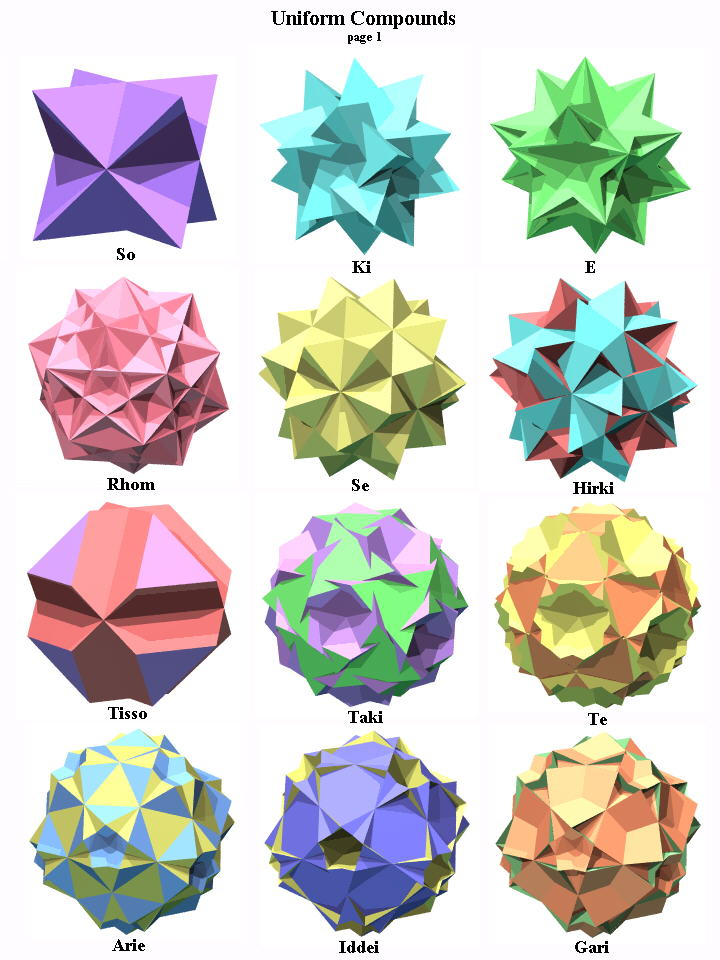```so (stella octangula) - S04 = self-dual compound of 2 tet within an encasing cube common intersection being an oct {4,3}[2{3,3}]{3,4} cf. details only flag-transitive 3D compound ki (chiro-icosahedron) - S05 = self-dual compound of 5 tet within an encasing doe common intersection being an ike {5,3}[5{3,3}]{3,5} e (icosiicosahedron) - S06 = self-dual compound of 10 tet within an encasing doe common intersection being an ike vertices coincide by pairs 2{5,3}[10{3,3}]2{3,5} rhom (rhombihedron) - S09 = compound of 5 cube edge-faceting of sidtid within an encasing doe common intersection being a rhote vertices coincide by pairs 2{5,3}[5{4,3}] se (small icosiicosahedron) - S17 = compound of 5 oct within an encasing id common intersection being an ike [5{3,4}]2{3,5} hirki (hemirhombichiro-icosahedron) - S18 = compound of 5 thah within an encasing id tisso (truncated stella octangula) - S54 = compound of 2 tut within an encasing q3o4x common intersection being an oct [2{;3;3}]{3,4} taki (truncated chiro-icosahedron) - S55 = compound of 5 tut common intersection being an ike [5{;3;3}]{3,5} te (truncated icosiicosahedron) - S56 = compound of 10 tut common intersection being an ike [10{;3;3}]2{3,5} arie (antirhombicosicosahedron) - S59 = compound of 5 co iddei (icosidisicosahedron) - S61 = compound of 5 oho gari (great antirhombicosahedron) - S60 = compound of 5 cho ```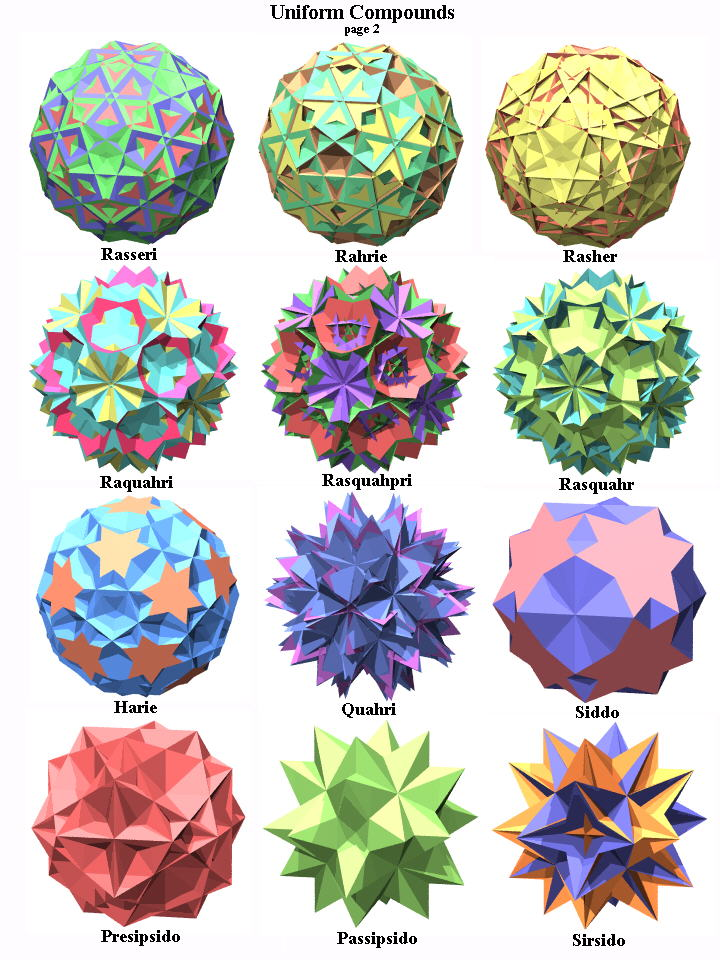```rasseri (rhombisnub rhombicosicoahedron) - S62 = compound of 5 sirco rahrie (rhombihyperhombicosicosahedron) - S64 = compound of 5 socco rasher (rhombisnub hyperhombihedron) - S63 = compound of 5 sroh raquahri (rhombiquasihyperhombicosicosahedron) - S65 = compound of 5 gocco rosaqri (rhombisnub quasirhombicosicosahedron) - S67 (old: rasquahpri = rhombisnub quasihyperpseudorhombicosicosahedron) = compound of 5 querco rasquahr (rhombisnub quasihyperrhombihedron) - S66 = compound of 5 groh tar (truncated rhombihedron) - S57 (old: harie = hyperhombicosicosahedron) = compound of 5 tic quitar (quasitruncated rhombihedron) - S58 (old: quahri = quasihyperhombicosicosahedron) = compound of 5 quith siddo (snub disoctahedron) - S46 = compound of 2 ike within an encasing x3f4o presipsido (pseudoretrosnub pentagonal snub (pseudo)disoctahedron) - S48 = compound of 2 gad within an encasing x3f4o passipsido (pseudosnub pentagrammatic snub (pseudo)disoctahedron) - S50 = compound of 2 sissid within an encasing x3f4o sirsido (small retrosnub disoctahedron) - S52 = compound of 2 gike within an encasing x3f4o ```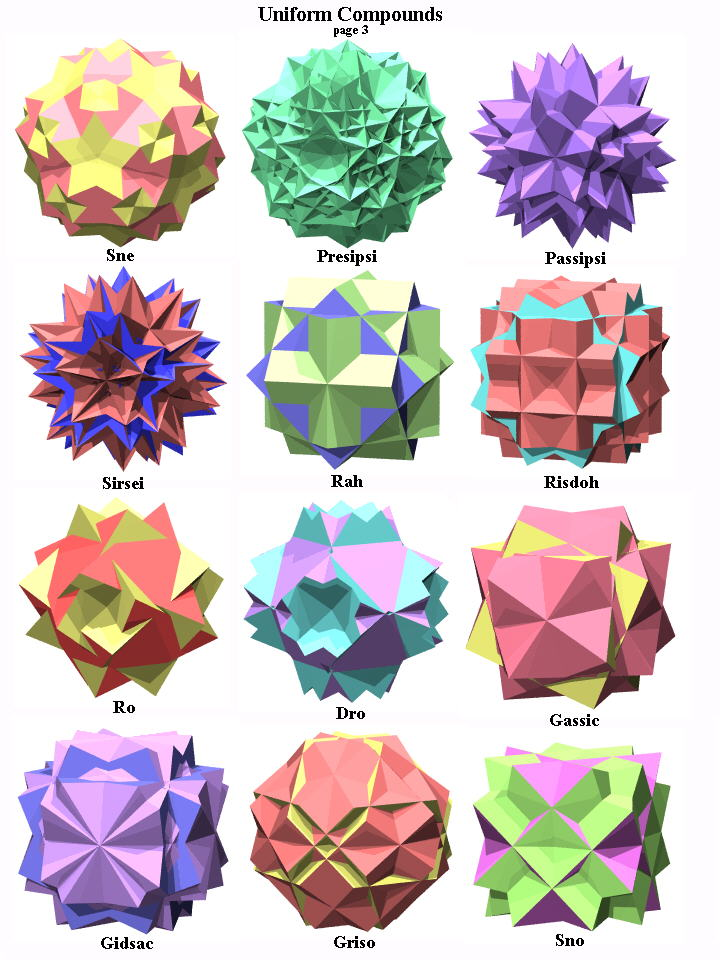```sne (snub icosiicosahedron) - S47 = compound of 5 ike presipsi (pseudoretrosnub pentagonal snub (pseudo)icosiicosahedron) - S49 = compound of 5 gad passipsi (pseudosnub pentagrammattic snub (pseudo)icosiicosahedron) - S51 = compound of 5 sissid sirsei (small retrosnub icosiicosahedron) - S53 = compound of 5 gike rah (rhombihexahedron) - S08 = compound of 3 cube within an encasing x3w4o common intersection being a sirco-stellation (the mere edge-truncation of the cube) risdoh (rhombisnub dishexahedron) - S07 = compound of 6 cube : rotational freedom ro ((chiral) rhombioctahedron) - S30 = compound of 4 trip dro (dirhombioctahedron) - S31 = compound of 8 trip gassic ((chiral) great snub cube) - S42 = compound of 3 squap gidsac (great disnub cube) - S43 = compound of 6 squap griso (great rhombisnub octahedron) - S38 = compound of 4 hip sno (snub octahedron) - S12 = compound of 4 oct within an encasing o3x4q ```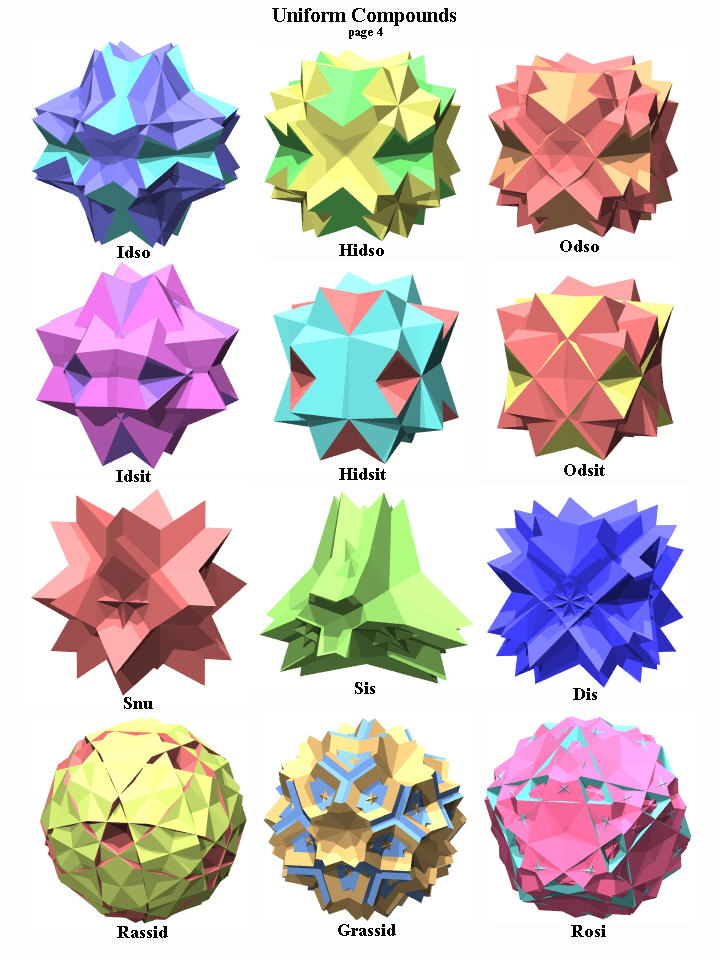```daso (old: doso; disnub octahedron) - S11 = compound of 8 oct : rotational freedom, see idso, hidso, odso idso (inner disnub octahedron) special range of daso hidso (hexagrammattic disnub octahedron) special case of daso (lateral {3} become (rotated) 2{3}-compounds) odso (outer disnub octahedron) special range of daso dissit (disnub tetrahedron) - S10 = compound of 4 oct : rotational freedom, see idsit, hidsit, odsit idsit (inner disnub tetrahedron) special range of dissit hidsit (hexagrammattic disnub tetrahedron) special case of dissit (lateral {3} become (rotated) 2{3}-compounds) odsit (outer disnub tetrahedron) special range of dissit sis (small snubihedron) - S01 = compound of 6 tet : rotational freedom, see snu snu (snubihedron) - S03 special case of sis (2-fold axes become 4-fold) within an encasing x3w4o dis (disnubihedron) - S02 = compound of 12 tet : rotational freedom rassid (rhombisnub dodecahedron) - S40 = compound of 6 dip grassid (great rhombisnub dodecahedron) - S41 = compound of 6 stiddip rosi (rhombisnub icosahedron) - S39 = compound of 10 hip ```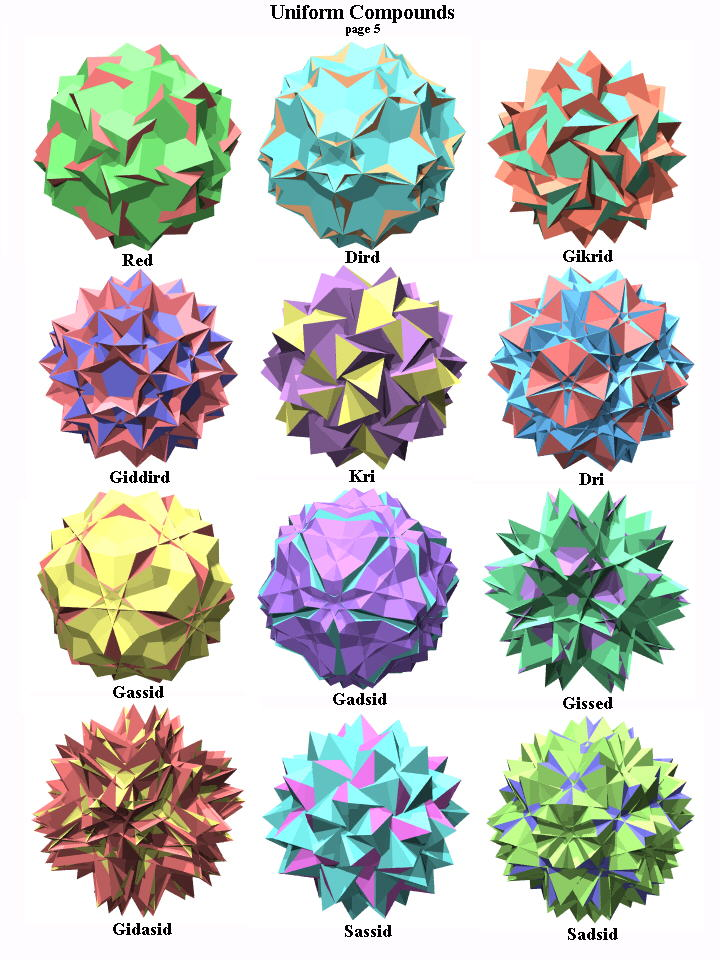```kred (old: red, chiro-rhombidodecahedron) - S34 = compound of 6 pip within an encasing f3x5o dird (dirhimbidodecahedron) - S35 = compound of 12 pip within an encasing f3x5o vertices coincide by pairs gikrid (great chirorhombidodecahedron) - S36 = compound of 6 stip within an encasing srid {;3,5;}[6{;5/2,2;}] giddird (great dirhombidodecahedron) - S37 = compound of 12 stip within an encasing srid vertices coincide by pairs 2{;3,5;}[12{;5/2,2;}] kri (chirorhombicosahedron) - S32 = compound of 10 trip within an encasing x3f5o dri (dirhombicosahedron) - S33 = compound of 20 trip within an encasing x3f5o vertices coincide by pairs gassid (great snub dodecahedron) - S27 = compound of 6 pap gadsid (great disnub dodecahedron) - S26 = compound of 12 pap : rotational freedom gissed (great invertisnub dodecahedron) - S29 = compound of 6 starp gidasid (great invertidisnub dodecahedron) - S28 = compound of 12 starp : rotational freedom sassid ((chiral) small snub dodecahedron) - S44 = compound of 6 stap sadsid (small disnub dodecahedron) - S45 = compound of 12 stap ```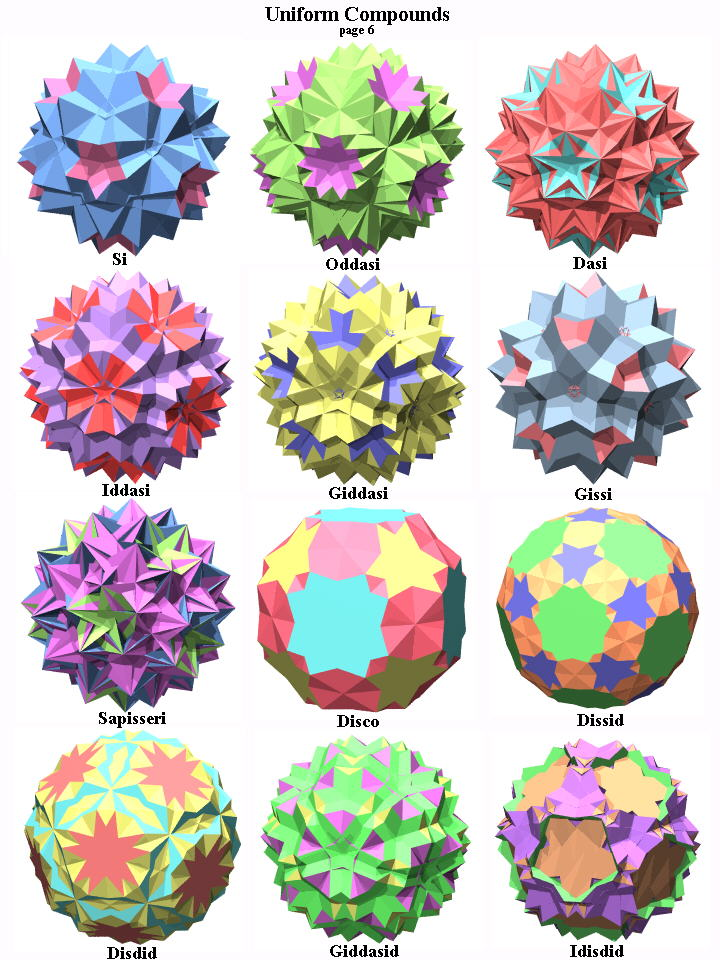```si (snub icosahedron) - S16 = compound of 10 oct addasi (altered disnub icosahedron) - S13 : rotational freedom, see oddasi, dasi, iddasi, giddasi oddasi (outer disnub icosahedron) = compound of 20 oct special range of addasi dasi (disnub icosahedron) - S14 = compound of 20 oct special case of addasi edge-faceting of gidrid vertices coincide by pairs iddasi (inner disnub icosahedron) = compound of 20 oct special range of addasi giddasi (great disnub icosahedron) = compound of 20 oct special range of addasi gissi (great snub icosahedron) - S15 = compound of 10 oct sapisseri ((chiral) snub pseudosnub rhombicosahedron) - S19 = compound of 20 thah edge-faceting of gidrid vertices coincide by pairs disco (disnub cuboctahedron) - S68 = compound of 2 snic dissid (disnub icosidodecahedron) - S69 = compound of 2 snid disdid (disnub dodecadodecahedron) - S73 = compound of 2 siddid giddasid (great disnub icosidodecahedron) - S70 = compound of 2 gosid idisdid (invertidisnub dodecadodecahedron) - S74 = compound of 2 isdid ```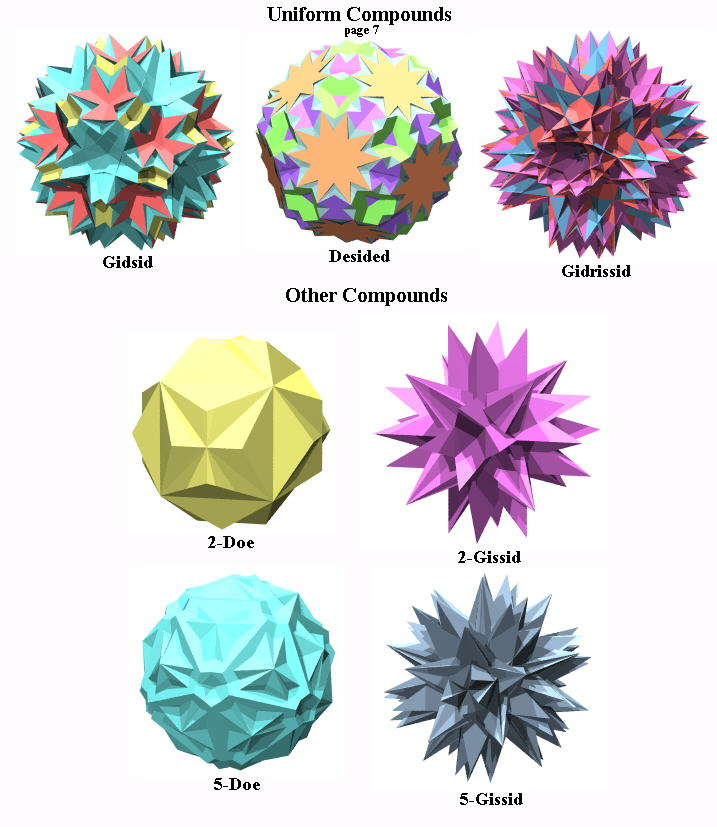```gidsid (great invertidisnub icosidodecahedron) - S71 = compound of 2 gisid desided (disnub icosidodecadodecahedron) - S75 = compound of 2 sided gidrissid (great diretrosnub icosidodecahedron) - S72 = compound of 2 girsid ```

The similar compound of 2 enantiomorphic snubs (as given on Bowers last 2 figure plates above) for the case of gisdid there (and usually) is excluded from the listings for the same reason as Grünbaumian figures were excluded from normal polytopes, i.e. because of the complete coincidence of facial elements (pentagrams in that case). That one would be a further edge faceting of gidrid. – Likewise the compound of 3 (orthogonal) op is here not contained. The corresponding blend is nothing but sroh. Conjungately the compound of 3 (orthogonal) stop, blending out the coincident squares, would result in groh.

None the same, what really is missing in these above pictures, are all the axial cases, based on the 2D compounds cf.Axials n/d-prisms n/d-antiprismsd odd n/d-antiprismsd even regularcompoundbasesS21 (esp. 2 cubes)S23 (esp. 2 oct)S25 rotationalfreedomS20S22S24

Interesting compounds with higher symmetry than that of its componenents so can even be obtained from non-uniform components. Sure, the result then will not become uniform either. As an according example might serve the compound of 5 rad.

```©
```

```
----
2D  (euclidean tilings)
----
```

At the end of 2020 Binnendyk came up with some compounds of flat euclidean tilings as well. He found them by generalizing common edge figures of spherical space polyhedra or according compounds to higher numbers, then becoming flat. (© : The below provided hand-drawn sketches all are his and are reproduced from that cited page.)

vertex
configuration
P = 4
(spherical)
P = 5
(spherical)
P = 6
(euclidean compound)
(3, 6, P/2, 6)
```©
```
tisso = 2-tut-compound
```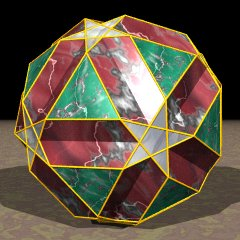©
```
siid
``````
2-that-compound
(P/2, P, P/2, P)
```
```
compound of 3
square-dihedra
(as diagonals of oct)
together with inserted
Grünbaumian
edge multicovers
```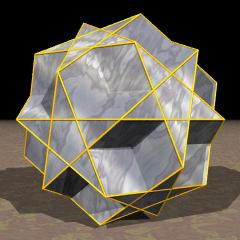©
```
did
``````
3-that-compound
(3, 2P/3, P, 2P/3)
```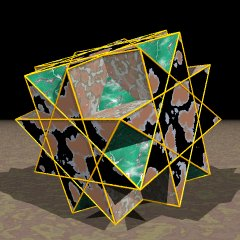©
```
gocco
```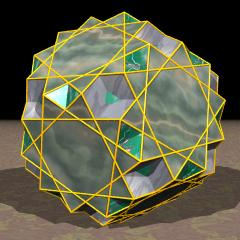©
```
gidditdid
``````
4-rothat-compound
(4, P/2, 4, P)
```©
```
rah = 3-cube-compound
```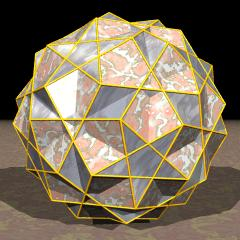©
```
```©
```
3-rothat-compound
(6, 2P, 2P/3)
```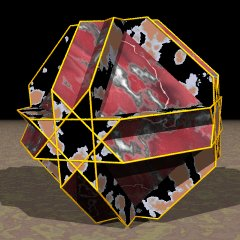©
```
cotco
```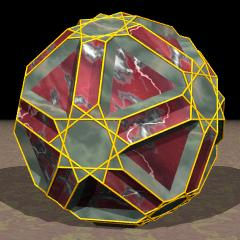©
```
idtid
```©
```
4-grothat-compound
(2P/3, 2P/3, (10-P)/(6-P))
```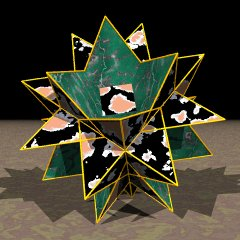©
```
quith
```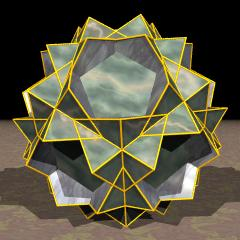©
```
quit sissid
``````
(dense) ∞-azip-compound
(4, 2P/3, 2(10-P)/(6-P))
```©
```
quitco
```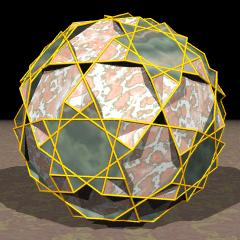©
```
quitdid
``````
(sparse) ∞-azip-compound

```
----
4D
----
```

Because the set of uniform polychora is not proven to be enlisted completely so far, even less is known about uniform compounds of 4-dimensional space. And even of those which are known so far only some are listed here ...

sorted alphabetically by components
```teppix  = compound of 60 deca
in dattady regiment, in hi army
vertices coincide by 3
3{5,3,3}[60{3;3;3}]

???     = compound of 6 dedip
in hi army
{5,3,3}[6{;5,2;5}]

sadtap  = compound of 36 dedip
in sidtaxhi regiment, in hi army
vertices coincide by 6, edges coincide by pairs
6{5,3,3}[36{;5,2;5}]

fasp    = compound of 12 distadedip
("fissal swirlprism")

xehix   = compound of 60 duhd
("hexacosahemihexacosachoron")
in sishi regiment

katdex  = compound of 2 ex
("chirotetrahedral dishexacosachoron")

spohi   = compound of 5 ex
in hi army
{5,3,3}[5{3,3,5}]

kepisna = compound of 6 ex
in rox army
{3;3,5}[6{3,3,5}]

sody    = compound of 10 ex
in hi army
vertices coincide by pairs
2{5,3,3}[10{3,3,5}]

kidisna = compound of 10 ex
in rahi army
{3,3;5}[10{3,3,5}]

pedisna = compound of 12 ex
in rox army
vertices coincide by pairs
2{3;3,5}[12{3,3,5}]

ditusna = compound of 20 ex
in rahi army
vertices coincide by pairs
2{3,3;5}[20{3,3,5}]

firmax  = compound of 25 firp
("facetorectified medial hexacosachoron")
in romex regiment

frahdet = compound of 2 firt

fispohi (old: fasophi) = compound of 5 fix
("faceted small pentishecatonicosachoron")
in hi army
{5,3,3}[5{3,5,5/2}]

kihp    = compound of 6 fix
("chiroicosahedral hyperprismatochoron")
in rox army

fassody = compound of 10 fix
("faceted small deckishecatonicosachoron")
in hi army
vertices coincide by pairs
2{5,3,3}[10{3,5,5/2}]2{3,3,5}

dihp    = compound of 12 fix
("disicosahedral hyperprismatochoron")
in rox army
vertices coincide by pairs

firdox (old: fapchix) = compound of 25 frico
("facetorectified dodecahedronary-hexacosachoron",
old: "facetopentacubic hecatonicosahexacosachoron")
in rissidtixhi regiment, in rahi army

gogakix = compound of 5 gaghi
("great grand chirohexacosachoron")
in hi army
{5,3,3}[5{5,5/2,3}]

gigakidhep = compound of 6 gaghi
("great grand chirododecahedral hyperprismatochoron")
in rox army

gigadex = compound of 10 gaghi
("great grand dishexacosachoron")
in hi army
vertices coincide by pairs
2{5,3,3}[10{5,5/2,3}]2{3,3,5}

gigadidhep = compound of 12 gaghi
("great grand disdodecahedral hyperprismatochoron")
in rox army
vertices coincide by pairs

gakix   = compound of 5 gahi
("grand chirohexacosachoron")
in hi army
{5,3,3}[5{5,3,5/2}]

gakidhep = compound of 6 gahi
("grand chirododecahedral hyperprismatochoron")
in rox army

gadex   = compound of 10 gahi
("grand dishexacosachoron")
in hi army
vertices coincide by pairs
2{5,3,3}[10{5,3,5/2}]2{3,3,5}

gadidhep = compound of 12 gahi
("grand disdodecahedral hyperprismatochoron")
in rox army
vertices coincide by pairs

gaqragi = compound of 3 gaqrit
("great quasirhombated gico")

gaqrigastic = compound of 6 gaqrit
("great quasirhombated gistic")

gaskix  = compound of 5 gashi
("grand stellated chirohexacosachoron")
in hi army
{5,3,3}[5{5/2,5,5/2}]

("grand stellated chirododecahedral hyperprismatochoron")
in rox army

gasdex  = compound of 10 gashi
("grand stellated dishexacosachoron")
in hi army
vertices coincide by pairs
2{5,3,3}[10{5/2,5,5/2}]2{3,3,5}

gasdadhep = compound of 12 gashi
("grand stellated disdodecahedral hyperprismatochoron")
in rox army
vertices coincide by pairs

gasspohi = compound of 5 gax
("grand snub pentishecatonicosachoron")
in hi army
{5,3,3}[5{3,3,5/2}]

gassody = compound of 10 gax
("grand snub deckishecatonicosachoron")
in hi army
vertices coincide by pairs
2{5,3,3}[10{3,3,5/2}]

gakpisna = compound of 6 gax
("grand chiropental snubbachoron")
in rox army

gapdisna = compound of 12 gax
("grand pental disnubbachoron")
in rox army
vertices coincide by pairs

afpox   = compound of 60 gippid
in affixthi regiment
vertices coincide by pairs

gepdi   = compound of 3 girdo
("great prismatodisicositetrachoron")
in giddic regiment, in spic army

giskix  = compound of 5 gishi
("great stellated chirohexacosachoron")
in hi army
{5,3,3}[5{5/2,3,5}]

giskadhep = compound of 6 gishi
("great stellated chirododecahedral hyperprismatochoron")
in rox army

gisdex  = compound of 10 gishi
("great stellated dishexacosachoron")
in hi army
vertices coincide by pairs
2{5,3,3}[10{5/2,3,5}]2{3,3,5}

gisdadhep = compound of 12 gishi
("great stellated disdodecahedral hyperprismatochoron")
in rox army
vertices coincide by pairs

gisdidhop = compound of 20 gishi
("great stellated disicosahedronary dishyperprismatochoron")

kiparp  = compound of 6 gisp
("chiropentagrammatic retroprismatochoron")
in rox army

diparp  = compound of 12 gisp
("dipentagrammatic retroprismatochoron")
in rox army
vertices coincide by pairs

gifspohi = compound of 5 gofix
("great faceted small pentishecatonicosachoron")
in hi army
{5,3,3}[5{3,5/2,5}]

gikihp  = compound of 6 gofix
("great chiroicosahedral hyperprismatochoron")
in rox army

gifsody = compound of 10 gofix
("great faceted small deckishecatonicosachoron")
in hi army
vertices coincide by pairs
2{5,3,3}[10{3,5/2,5}]2{3,3,5}

gidihp  = compound of 12 gofix
("great disicosahedral hyperprismatochoron")
in rox army
vertices coincide by pairs

gogiskix = compound of 5 gogishi
("great grand stellated chirohexacosachoron")

("great grand stellated chirododecahedral hyperprismatochoron")
in f3o3x5o army

gogisdex = compound of 10 gogishi
("great grand stellated dishexacosachoron")

gogasdadhep = compound of 12 gogishi
("great grand stellated disdodecahedral hyperprismatochoron")
in f3o3x5o army

gokix   = compound of 5 gohi
("great chirohexacosachoron")
in hi army
{5,3,3}[5{5,5/2,5}]

gikadhep = compound of 6 gohi
("great chirododecahedral hyperprismatochoron")
in rox army

godex   = compound of 10 gohi
("great dishexacosachoron")
in hi army
vertices coincide by pairs
2{5,3,3}[10{5,5/2,5}]2{3,3,5}

gedadhep = compound of 12 gohi
("great disdodecahedral hyperprismatochoron")
in rox army
vertices coincide by pairs

gedidhop = compound of 20 gohi
("great disicosahedronary dishyperprismatochoron")

garstic = compound of 2 grico
("great rhombated stellated tetracontoctachoron")

gristed = compound of 2 (inverted) grip
("great rhombated stellated decachoron")

gargi   = compound of 3 grit
("great rhombated gico")

gargastic = compound of 6 grit
("great rhombated gistic")

ditsop  = compound of 12 gudap
("ditrigonary swirlprism")
in sishi regiment, in ex army

haddet  = compound of 2 hex
("demidistesseract")
in tes army
{4,3,3}[2{3,3,4}]

stico    = compound of 3 hex
in ico army
{3,4,3}[3{3,3,4}]2{3,4,3}
flag-transitive

sistic  = compound of 6 hex
("stellated stoc")
in stoc army
flag-transitive

kixfig  = compound of 8 hex
("chirocrossifissal 64-choron")
in odip army
(because hex is just the 8-3-stepprism within odip)
chiral

kotapna = compound of 12 hex
("chirotrigonal antiprismary enenahexachoron")
in rico army
{3;4,3}[12{3,3,4}]

cefdig  = compound of 16 hex
("crossifissal dis64-choron")
in odip army
(because hex is just the 8-3-stepprism within odip)

botapna = compound of 24 hex
("bitrigonal antiprismary enenahexachoron")
in rico army
vertices coincide by pairs
2{3;4,3}[24{3,3,4}]

hesatdi = compound of 50 hex
(obtained as alternation of each tes
in the 25 tes compound)

stidox  = compound of 75 hex
in ex army
vertices coincide by 5
5{3,3,5}[75{3,3,4}]10{5,3,3}
= compound of 25 stico

???     = compound of 675 hex
in hi army
vertices coincide by 9
9{5,3,3}[675{4,3,3}]18{3,3,5}

kadhep  = compound of 6 hi
("chirododecahedral hyperprismatochoron")
in f3o3x5o army

dadhep  = compound of 12 hi
("disdodecahedral hyperprismatochoron")
in f3o3x5o army

affip   = compound of 16 hiddip
in afdec regiment, in cont army
vertices coincide by pairs
2{3,4,3}[16{;6,2;6}]

datap   = compound of 100 hiddip
in dattady regiment, in hi army
vertices coincide by 6, edges coincide by pairs
6{5,3,3}[100{;6,2;6}]

dethnah = compound of 2 hinnit

stoc    = compound of 2 (dual) ico
in bicont (cont-dual) army
common intersection being a cont
[2{3,4,3}]{3;4;3}
flag-transitive

kitapna = compound of 4 ico
in rico army
{3;4,3}[4{3,4,3}]

chi     = chiral compound of 5 ico
in a chiral sub-sishi regiment, in ex army
(not all edges being used)
common intersection being a hi
{3,3,5}[5{3,4,3}]{5,3,3}

kitefa  = compound of 6 ico
("chirotegmic hecatontetracontatetrachoron")
in spic army
{;3,4,3;}[6{3,4,3}]

bitapna = compound of 8 ico
in rico army
vertices coincide by 2
2{3;4,3}[8{3,4,3}]

bitefa  = compound of 12 ico
("bitegmic hecatontetracontatetrachoron")
in spic army
2{;3,4,3;}[12{3,4,3}]

???     = compound of 20 ico
in a chiral sub-sishi regiment, in ex army
= dox - chi

dox     = compound of 25 ico
in sishi regiment, in ex army
common intersection being an ex
vertices coincide by 5
5{3,3,5}[25{3,4,3}]{3,3,5}

???     = compound of 225 ico
in hi army
vertices coincide by 9
9{5,3,3}[225{3,4,3}]

???     = dual compound of 225 ico
common intersection being an ex
9 octs each are co-realmic (building a compound on their own)
[225{3,4,3}]9{3,3,5}

sadixhix = compound of 25 ihi
in sishi regiment, in ex army
vertices coincide by 5
(different orientation of ihis than for gadixhix)

gadixhix = compound of 25 ihi
in sishi regiment, in ex army
vertices coincide by 5
(different orientation of ihis than for sadixhix)

xix     = compound of 25 ini
("hexacosahexacosachoron")
in rissidtixhi regiment, in rahi army

???     = [Grünbaumian] compound of 3 odip
in srit regiment
vertices coincide by 2
blending out pairs of coincident squares → garpit

???     = [Grünbaumian] compound of 9 odip
in spic regiment
vertices coincide by 4
blending out pairs of coincident squares → sirc

ostople = compound of 2 ostodip
({8}x{8/3} + {8/3}x{8})

```
```pedeple = compound of 2 padedip
({5}x{10} + {10}x{5})

gakskos = compound of 5 paphacki
("great chirosnub chillioctacosachoron")

???     = compound of 10 paphacki
in hi army
in 10-sishi-compound regiment

???     = compound of 10 paphicki
in hi army
in 10-sishi-compound regiment

sadegdip = compound of 4 pedip
("stellated-decagonal duoprism")
in dedip army
{10}x{10}[4{5}x{5}]

tripendip = compound of 9 pedip
("tripentagonal duoprism")
{15}x{15}[9{5}x{5}]

???     = compound of 16 pedip
{20}x{20}[16{5}x{5}]

sted    = compound of 2 (dual) pen
in bideca (deca-dual) army
common intersection being a deca
[2{3,3,3}]{3;3;3} cf. details
flag-transitive

gysfi   = compound of 4 pen
("gyrosimplexifissal icosachoron")
in pedip army
chiral

kisfis  = compound of 5 pen
("chirosimplexifissal icosipentachoron")
in pedip army
(because pen is just the 5-2-stepprism within pedip)
chiral

sifdis  = compound of 10 pen
("simplexifissal disicosipentachoron")
in pedip army

???     = compound of 20 pen
in hi army
{5,3,3}[20{3,3,3}]

mix     = compound of 120 pen
in mix regiment, in hi army
common intersection being an ex
{5,3,3}[120{3,3,3}]{3,3,5}
flag-transitive

???     = compound of 720 pen
in hi army
6{5,3,3}[720{3,3,3}]

pinnix  = compound of 120 pinnip
("prismatointercepted hexacosachoron")
in romex regiment

pestideple = compound of 2 pistadedip
({5}x{10/3} + {10/3}x{5})

gecupady = compound of 5 prarsi
("great cupoliprismatodishecatonicosachoron")

parstic  = compound of 2 prico
("prismatorhombated stellated tetracontoctachoron")

pirsted = compound of 2 (inverted) prip
("prismatorhombated stellated decachoron")

scupady = compound of 5 prissi
("small cupoliprismatodishecatonicosachoron")

pregi   = compound of 3 prit
("prismatorhombated gico")

pirgastic = compound of 6 prit
("prismatorhombated gistic")

badhidy = non-isohedral compound of quit sishi and tigaghi

quitagi = compound of 3 quitit
("quasitruncated gico")

quit gistic = compound of 6 quitit
("quasitruncated gistic")

???     = compound of 10 raggix

risted  = compound of 2 (inverted) rap
("rectified stellated decachoron")

romex   = compound of 120 rap
("rectified medial hexacosachoron")
in romex regiment

rastic  = compound of 2 rico
("rectified stellated tetracontoctachoron")
rectified stoc

roktefa = compound of 6 rico
("rectified chirotegmic hecatontetraconttetrachoron")
rectified kitefa

robtefa = compound of 12 rico
("rectified bitegmic hecatontetraconttetrachoron")
rectified bitefa

redox (old: pichix) = compound of 25 rico
("rectified dodecahedronary-hexacosachoron",
old: "pentacubic hecatonicosahexacosachoron")
in rissidtixhi regiment, in rahi army
2{3,3;5}[25{3;4,3}]

brahdet = compound of 2 rit

ragi    = compound of 3 rit
("rectified gico")

ragistic = compound of 6 rit
("rectified gistic")

redac   = compound of 75 rit
("rectified dac")
in rahi army

rekatdex  = compound of 2 rox
("rectified chirotetrahedral dishexacosachoron")

raspohi = compound of 5 rox
("rectified small pentishecatonicosachoron")

???     = compound of 10 rox

spagi   = compound of 3 sidpith
("small prismated gico")

spagastic = compound of 6 sidpith
("small prismated gistic")

sepdi   = compound of 3 sirdo
("small prismatodisicositetrachoron")
in spic regiment and army

siskix  = compound of 5 sishi
("small stellated chirohexacosachoron")
in hi army
{5,3,3}[5{5/2,5,3}]

skadhep = compound of 6 sishi
("stellated chirododecahedral hyperprismatochoron")
in rox army

sisdex  = compound of 10 sishi
("small stellated dishexacosachoron")
in hi army
vertices coincide by pairs
2{5,3,3}[10{5/2,5,3}]2{3,3,5}

sidadhep = compound of 12 sishi
("stellated disdodecahedral hyperprismatochoron")
in rox army
vertices coincide by pairs

kepap   = compound of 6 sisp
("chiropentagonal antiprismatochoron")
in rox army

dipap   = compound of 12 sisp
("dipentagonal antiprismatochoron")
in rox army
vertices coincide by pairs

sistople = compound of 2 sistodip
in gittith regiment, in tat army
({4}x{8/3} + {8/3}x{4})

sople   = compound of 2 sodip
in sidpith regiment and army
({4}x{8} + {8}x{4})

dopix   = compound of 60 spid
in sishi regiment, in ex army
vertices coincide by 10, edges coincide by 3
10{3,3,5}[60{;3,3,3;}]

???     = compound of 12 spidrox
in o3f3x5o army

serstic = compound of 2 srico
("small rhombated stoc")

sristed = compound of 2 (inverted) srip
("small rhombated stellated decachoron")

???     = compound of 3 srit
in spic regiment
vertices coincide by 2
2{;3,4,3;}[3{3;3,4;}]

vertices coincide by 6, edges coincide by pairs
6{5,3,3}[36{;10/3,2;10/3}]

stardeple = compound of 2 stardedip
({5/2}x{10} + {10}x{5/2})

starpeple = compound of 2 starpedip
({5}x{5/2} + {5/2}x{5})

starpepla = compound of 2 starpedip
({5}x{5/2} + inv{5/2}x inv{5})

???     = compound of 4 starpedip
in gap army

spidy   = compound of 24 starpedip
in sishi regiment, in ex army
vertices coincide by 5
5{3,3,5}[24{;5,2;5/2}]

starstideple = compound of 2 stastidedip
({5/2}x{10/3} + {10/3}x{5/2})

???     = [Grünbaumian] compound of 3 stodip
in wavitoth regiment
vertices coincide by 2
blending out pairs of coincident squares → gaqript

???     = [Grünbaumian] compound of 9 stodip
in giddic regiment
vertices coincide by 4
blending out pairs of coincident squares → girc

bitagi  = compound of 3 tah
("bitruncated gico")

butgastic = compound of 6 tah
("bitruncated gistic")

tagi    = compound of 3 tat
("truncated gico")

tagistic = compound of 6 tat
("truncated gistic")

gico    = compound of 3 tes
in ico regiment
common intersection being an ico
vertices coincide by pairs
2{3,4,3}[3{4,3,3}]{3,4,3}
flag-transitive

socdip  = compound of 4 tes
in odip army
{8}x{8}[4{4,3,3}]

gistic  = compound of 6 tes
("great stellated icositetrachoron")
in stoc army
= compound of 2 gico
flag-transitive

trisqdip = compound of 9 tes
{12}x{12}[9{4,3,3}]

kitcana = compound of 12 tes
("chirotrigonal crystallic enenahexachoron")
in rico army
vertices coincide by pairs
2{3;4,3}[12{4,3,3}]

cac     = compound of 15 tes
("chirocubichoron")
in a chiral sub-sishi regiment, in ex army
(not all edges being used)
2{3,3,5}[15{4,3,3}]

tesqdip = compound of 16 tes
("tetrasquare duoprism")
{16}x{16}[16{4,3,3}]

bitcana = compound of 24 tes
("bitrigonal crystallic enenahexachoron")
in rico army
vertices coincide by 4
4{3;4,3}[24{4,3,3}]

???     = compound of 25 tes
{20}x{20}[25{4,3,3}]

???     = compound of 36 tes
{24}x{24}[36{4,3,3}]

???     = compound of 49 tes
{28}x{28}[49{4,3,3}]

???     = compound of 64 tes
{32}x{32}[64{4,3,3}]

dac     = compound of 75 tes
("dodecahedronary cubichoron")
in sishi regiment, in ex army
2{3,3,5}[75{4,3,3}]

???     = compound of 81 tes
{36}x{36}[81{4,3,3}]

???     = compound of 100 tes
{40}x{40}[100{4,3,3}]

???     = compound of 675 tes
in hi army
vertices coincide by 18
18{5,3,3}[675{4,3,3}]9{3,3,5}

tikatdex  = compound of 2 tex
("truncated chirotetrahedral dishexacosachoron")

tispohi = compound of 5 tex
("truncated small pentishecatonicosachoron")

tissody = compound of 10 tex
("truncated small deckishecatonicosachoron")

thiple  = compound of 2 thiddip
({3}x{6} + {6}x{3})

dathah  = compound of 2 tho
in tes army

chehah  = compound of 2 tho
in tes army
(different mutual orientation)

tastic  = compound of 2 tico
truncated stoc

tiktefa = compound of 6 tico
("truncated chirotegmic hecatontetraconttetrachoron")
truncated kitefa

tibtefa = compound of 12 tico
("truncated bitegmic hecatontetraconttetrachoron")
truncated bitefa

tidox   = compound of 25 tico
in tissidtixhi regiment
vertices coincide by pairs

tisted  = compound of 2 tip
truncated sted

tomex   = compound of 120 tip
truncated mix

shigdip = compound of 4 triddip
("stellated-hexagonal duoprism")
in hiddip army
{6}x{6}[4{3}x{3}]

fendip  = compound of 9 triddip
("fissary-enneagonal duoprism")
in edip army
{9}x{9}[9{3}x{3}]

tetridip = compound of 16 triddip
("tetratrigon duoprism")
{12}x{12}[16{3}x{3}]

pentridip = compound of 25 triddip
("pentatrigon duoprism")
{15}x{15}[25{3}x{3}]

???     = compound of 36 triddip
{18}x{18}[36{3}x{3}]

...
```

```
----
5D
----
```

Only few is known here so far ...

sorted alphabetically by components
```todin = compound of 2 (alternate) hin

stade = compound of 2 (dual) hix
common intersection being a dot
[2{;3,3,3,3}]{3,3;3,3} cf. details
only 5D flag-transitive

taxt  = compound of 6 hix
("triaxis trigonism")
vertex figure of tree
vertices coincide by 2

bic   = compound of 10 squoct
in rat regiment
vertices coincide by 6
6{3;3,3,4}[10{;4,2;3,4}]

...
```

```
----
6D
----
```

Only few is known here so far ...

sorted alphabetically by components
```soctip = compound of 8 ax
("stellated-octagonal triprism")
{8}x{8}x{8}[8{4,3,3,3,3}]

tree = compound of 15 ax
("trishexacontapeton")
in brag regiment
vertices coincide by 6
edges coincide by 2
in fact its 180 pent facets form 60 gicope

trisqtip = compound of 27 ax
("trisquare triprism")
{12}x{12}x{12}[27{4,3,3,3,3}]

tesqtip = compound of 64 ax
("tetrasquare triprism")
{16}x{16}x{16}[64{4,3,3,3,3}]

???  = compound of 125 ax
{20}x{20}x{20}[125{4,3,3,3,3}]

ket  = compound of 36 dotip
("heptacontiditetracositriacontidipeton")
in mo regiment
vertices coincide by 20
20  o3o3o3o3o *c3x [36  o3o3x3o3o x]

gedak  = compound of 2 (alternate) hax

pok  = compound of 36 hixip
("prismatoheptacontidipeton")
in jak regiment
vertices coincide by 16

```
```stef = compound of 2 (dual) hop
common intersection being a fe
[2{;3,3,3,3,3}]{3,3;3;3,3} cf. details
only 6D flag-transitive

ta   = compound of 5 hop
{3,3;3,3,3}[5{;3,3,3,3,3}]

madek  = compound of 2 (inverted) jak

wa   = compound of 20 tetdip
in hax regiment
vertices coincide by 10

shigtip = compound of 8 trittip
("stellated-hexagonal triprism")
{6}x{6}x{6}[8{3}x{3}x{3}]

fentip = compound of 27 trittip
("fissary-enneagonal triprism")
{9}x{9}x{9}[27{3}x{3}x{3}]

tetritip = compound of 64 trittip
("tetratrigon triprism")
{12}x{12}x{12}[64{3}x{3}x{3}]

ye   = compound of 80 trittip
("heptacosiicosapeton")
in mo regiment
vertices coincide by 30

...
```

```
----
7D
----
```

Only few is known here so far ...

sorted alphabetically by components
```faq  = compound of 36 he
("fissal pentacosihebdomecontahexaexon")
in laq regiment
vertices coincide by 20

???  = compound of 135 hept
in lin army

???  = compound of 30 naq
in barz army

she  = compound of 2 oca
(dual position)
flag-transitive

sig  = compound of 8 oca
("simplexial hexacontatetraexon")
in hesa army

```
```saz  = compound of 16 oca
("simplexial hecatonicosioctaexon")
in hept army
{4,3,3,3,3,3}[16{3,3,3,3,3,3}]{3,3,3,3,3,4}
that one works for any Mersenne number dimension (D = 2k-1)

fisq = compound of 72 oca
("fissal simplexic pentacosihebdomecontahexaexon")
(full symmetric)
in lin army
flag-transitive

rush = compound of 2 roc
(inverted orientations)
in naq army

rassiz = compound of 16 roc
("rectified simplexial hecatonicosioctaexon")

deq  = compound of 72 roc
(full symmetric)
in naq army
it's the rectified fisq

...
```

```
----
8D
----
```

Only few is known here so far ...

sorted alphabetically by components
```???  = compound of 270 fy
in bay army

sib  = compound of 2 ene
(dual position)
flag-transitive

may  = compound of 1920 ene
in bay army
(all 17280 facets combine into 2160 sigs)
self-dual

say  = compound of 1920 ene
in bif army
(all 17280 facets remain oca)
self-dual
flag-transitive

...
```

##### Intersection Kernels of Facet-Regular Bi-Simplex Compounds

Simplices in general do miss the inversion symmetry. Accordingly the compound of a mutually dual simplex pair exists for any dimension. It obviously is flag-transitive in any dimension. Also those all are facet-regular compounds. In fact, the intersection kernel not only can be provided generally in its combinatrical shape, but well in its correct metric size as well. In dimensional sequence we have:

```0D: intersection kernel of         o         and         o         is         o         when scaled down by 1
1D: intersection kernel of         x         and         x         is         u         when scaled down by 2
2D: intersection kernel of        x3o        and        o3x        is        x3x        when scaled down by 3
3D: intersection kernel of       x3o3o       and       o3o3x       is       o3u3o       when scaled down by 4
4D: intersection kernel of      x3o3o3o      and      o3o3o3x      is      o3x3x3o      when scaled down by 5
5D: intersection kernel of     x3o3o3o3o     and     o3o3o3o3x     is     o3o3u3o3o     when scaled down by 6
6D: intersection kernel of    x3o3o3o3o3o    and    o3o3o3o3o3x    is    o3o3x3x3o3o    when scaled down by 7
7D: intersection kernel of   x3o3o3o3o3o3o   and   o3o3o3o3o3o3x   is   o3o3o3u3o3o3o   when scaled down by 8
8D: intersection kernel of  x3o3o3o3o3o3o3o  and  o3o3o3o3o3o3o3x  is  o3o3o3x3x3o3o3o  when scaled down by 9
9D: intersection kernel of x3o3o3o3o3o3o3o3o and o3o3o3o3o3o3o3o3x is o3o3o3o3u3o3o3o3o when scaled down by 10
```